# ggsoccer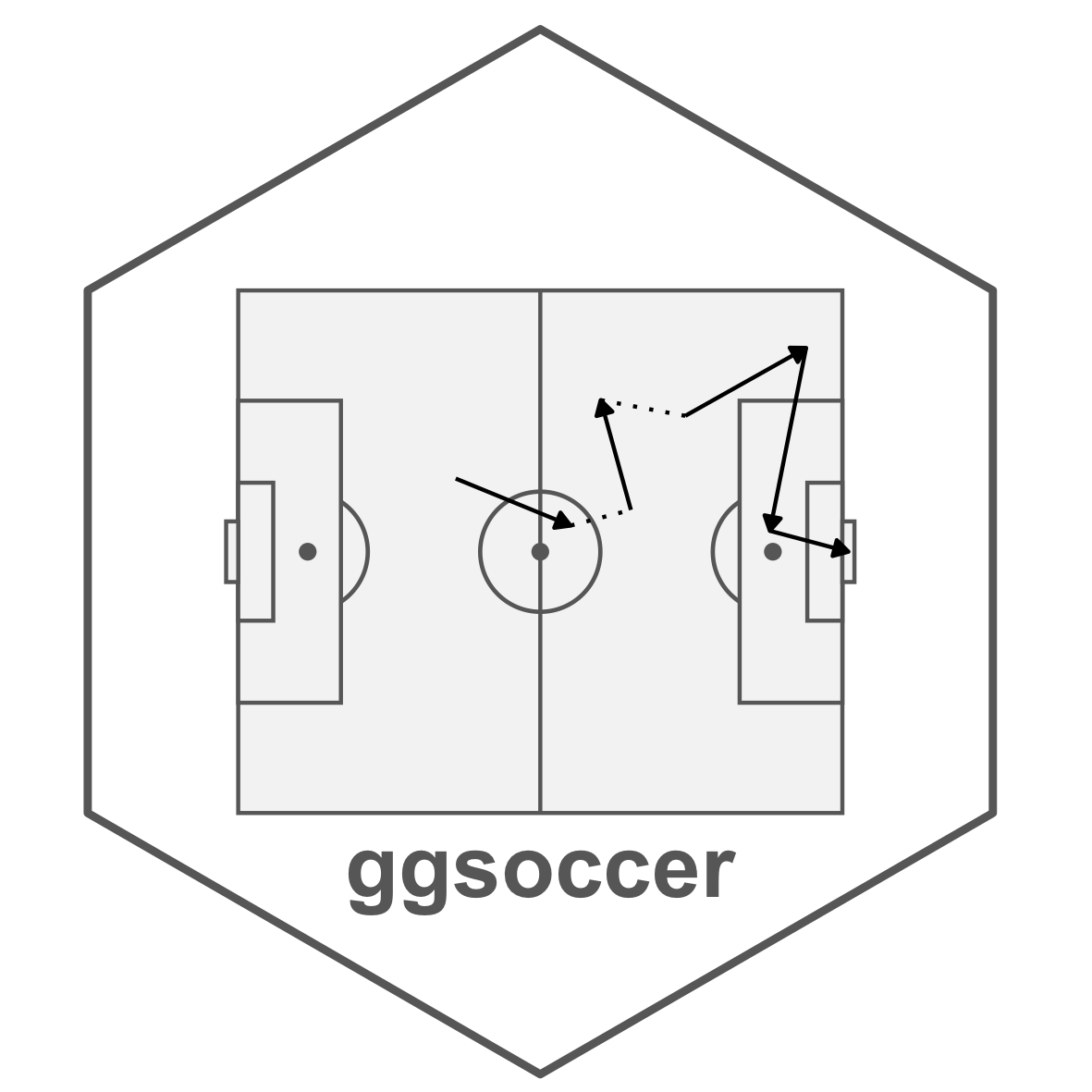## Overview

ggsoccer provides a functions for plotting soccer event data in R/ggplot2.

## Installation

ggsoccer is available via CRAN:

``install.packages("ggsoccer")``

``````# install.packages("remotes")
remotes::install_github("torvaney/ggsoccer")``````

## Usage

``````library(ggplot2)
library(ggsoccer)

ggplot() +
annotate_pitch() +
theme_pitch()``````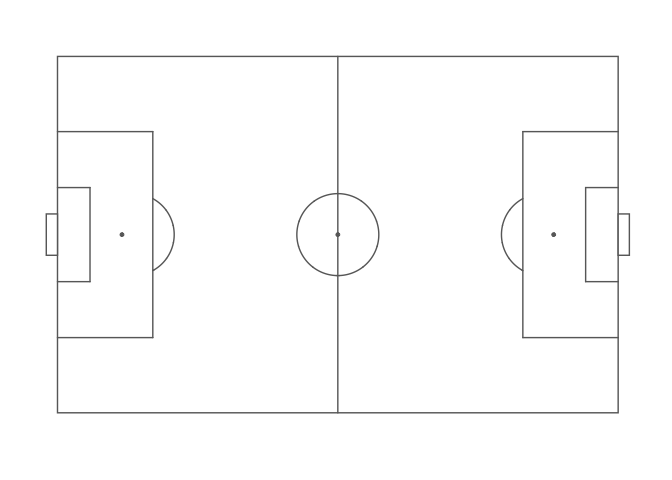The following example uses ggsoccer to solve a realistic problem: plotting a set of passes onto a soccer pitch.

``````pass_data <- data.frame(x = c(24, 18, 64, 78, 53),
y = c(43, 55, 88, 18, 44),
x2 = c(34, 44, 81, 85, 64),
y2 = c(40, 62, 89, 44, 28))

ggplot(pass_data) +
annotate_pitch() +
geom_segment(aes(x = x, y = y, xend = x2, yend = y2),
arrow = arrow(length = unit(0.25, "cm"),
type = "closed")) +
theme_pitch() +
direction_label() +
ggtitle("Simple passmap",
"ggsoccer example")``````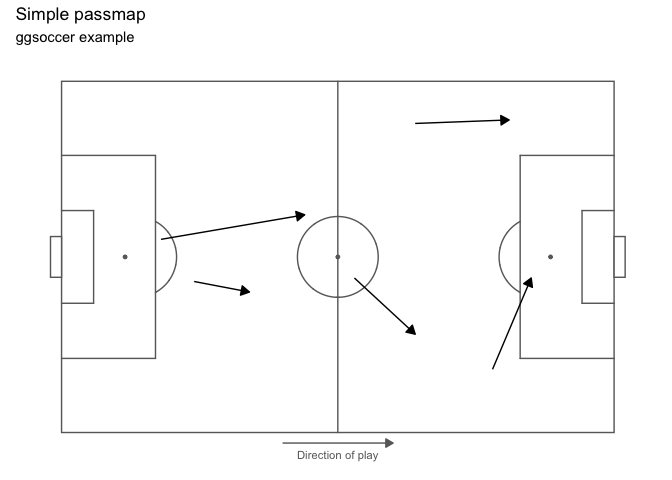Because ggsoccer is implemented as ggplot layers, plots can be customised with standard ggplot functions and layers.

Here is a different example, plotting shots on a green pitch.

By default, ggsoccer will display the whole pitch. To display a subsection of the pitch, set the plot limits as you would with any other ggplot2 plot. Here, we use the `xlim` and `ylim` arguments to `coord_flip`.

`coord_flip` reverses the orientation of the points, so we must also reverse the y-axis to ensure that the orientation remains correct (that is, shots from the left hand side appear on the left, and right-sided shots appear on the right).

You can do this with either `scale_y_reverse` or by reversing the order of the limits in `coord_flip`’s `ylim` argument.

If you don’t correct (i.e. reverse) the y axis orientation, the penalty box arcs will appear inside the box!

``````shots <- data.frame(x = c(90, 85, 82, 78, 83, 74, 94, 91),
y = c(43, 40, 52, 56, 44, 71, 60, 54))

ggplot(shots) +
annotate_pitch(colour = "white",
fill   = "springgreen4",
limits = FALSE) +
geom_point(aes(x = x, y = y),
colour = "yellow",
size = 4) +
theme_pitch() +
theme(panel.background = element_rect(fill = "springgreen4")) +
coord_flip(xlim = c(49, 101)) +
scale_y_reverse() +
ggtitle("Simple shotmap",
"ggsoccer example")``````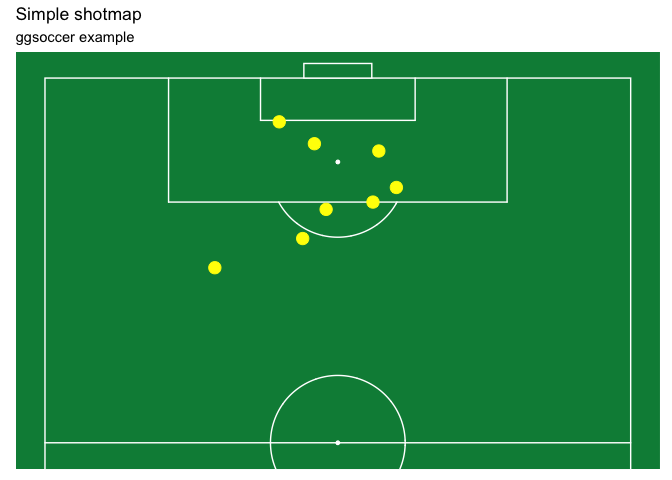### Data providers

ggsoccer defaults to Opta’s 100x100 coordinate system. However, different data providers may use alternative coordinates.

ggsoccer provides support for a few data providers out of the box, as well as an interface for any custom coordinate system:

• Opta
• Statsbomb
• Wyscout

#### Statsbomb

``````# ggsoccer enables you to rescale coordinates from one data provider to another, too
to_statsbomb <- rescale_coordinates(from = pitch_opta, to = pitch_statsbomb)

passes_rescaled <- data.frame(x  = to_statsbomb\$x(pass_data\$x),
y  = to_statsbomb\$y(pass_data\$y),
x2 = to_statsbomb\$x(pass_data\$x2),
y2 = to_statsbomb\$y(pass_data\$y2))

ggplot(passes_rescaled) +
annotate_pitch(dimensions = pitch_statsbomb) +
geom_segment(aes(x = x, y = y, xend = x2, yend = y2),
colour = "coral",
arrow = arrow(length = unit(0.25, "cm"),
type = "closed")) +
theme_pitch() +
direction_label(x_label = 60) +
ggtitle("Simple passmap",
"Statsbomb co-ordinates")``````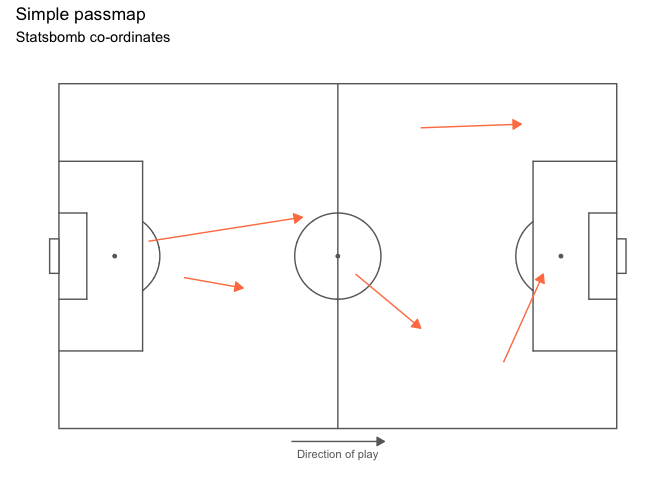#### Custom data

To plot data for a dataset not provided, ggsoccer requires a pitch specification. This is a list containing the required pitch dimensions like so:

``````pitch_custom <- list(
length = 150,
width = 100,
penalty_box_length = 25,
penalty_box_width = 60,
six_yard_box_length = 8,
six_yard_box_width = 26,
penalty_spot_distance = 16,
goal_width = 12,
origin_x = 0,
origin_y = 0
)

ggplot() +
annotate_pitch(dimensions = pitch_custom) +
theme_pitch()``````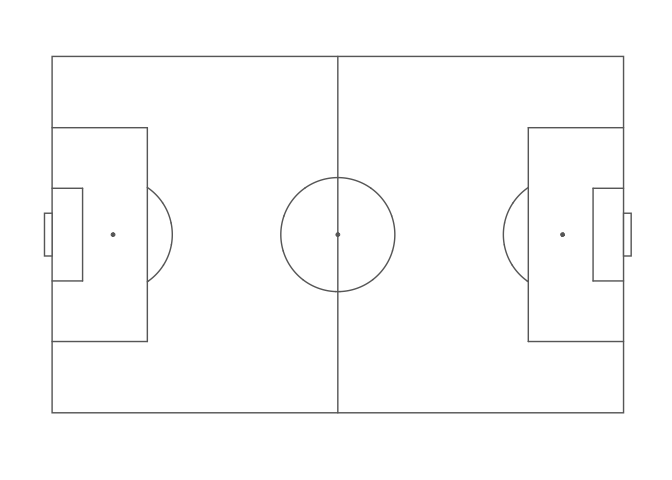### Goals

ggsoccer allows you to customise your goals markings by supplying a function to the `goals` argument of `annotate_pitch`:

``````ggplot() +
annotate_pitch(fill = "steelblue4", colour = "white", goals = goals_line) +
theme_pitch() +
theme(panel.background = element_rect(fill = "steelblue4"))``````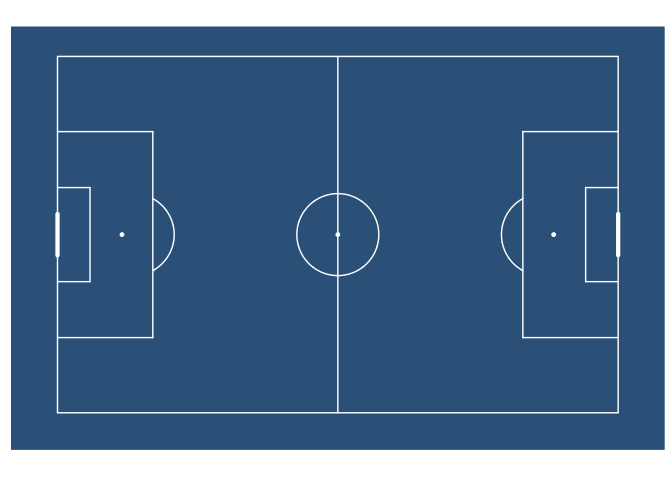``````ggplot() +
annotate_pitch(goals = goals_strip, fill = "lightgray") +
theme_pitch()``````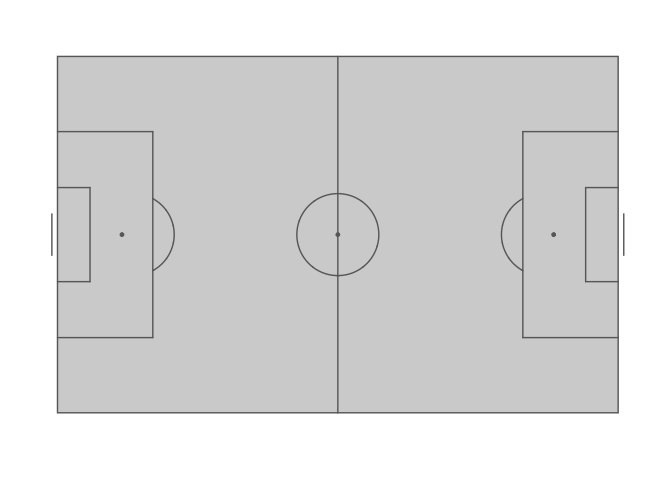This argument takes a function (or one-sided formula). You can use the supplied functions, or create your own goal markings function. The `goals` argument also supports using one-sided formulas as lambda functions (see `rlang::as_function`).

Custom goals functions must accept the arguments used by `annotate_pitch`: `colour`, `fill`, `dimensions`, `linewidth`, `alpha`, and `linetype`. Additional arguments can also be added.

``````goals_custom <- function(colour, fill, dimensions, ...) {
goals_strip(colour, fill, dimensions, lineend = "square", linewidth = 3.5)
}

ggplot() +
annotate_pitch(
goals = goals_custom,
fill = "lightgray"
) +
theme_pitch()``````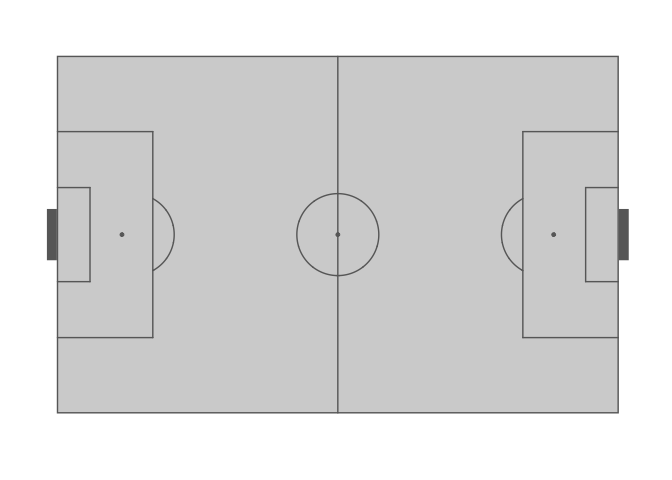See `help(goals_box)` for the full list of available functions.

The idea for having multiple goal markings was taken and adapted from the fc.rstats package.

### Further customisation

You can also alter the style of pitch markings with `linewidth`, `alpha`, and `linetype`:

``````ggplot() +
annotate_pitch(
colour = "white",
linewidth = 1.5,
linetype = "12",
alpha = 0.2,
goals = goals_line
) +
theme_pitch() +
theme(panel.background = element_rect(fill = "steelblue"))``````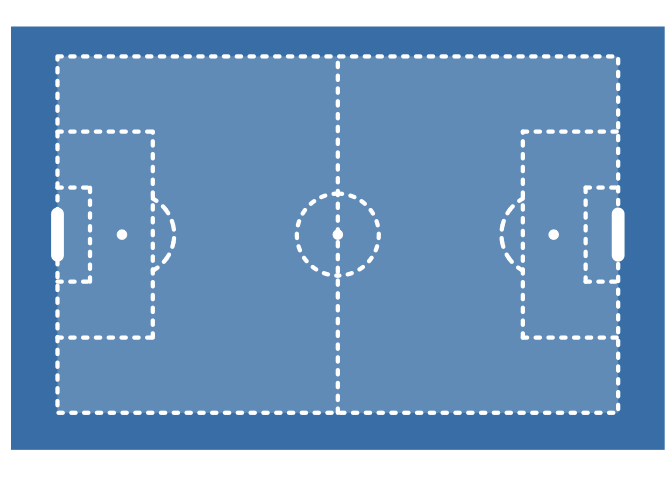## Other options

There are other packages that offer alternative pitch plotting options. Depending on your use case, you may want to check these out too:

### Python

There are a couple of pitch plotting options for matplotlib, too: